# The Rhombicuboctahedron

The rhombicuboctahedron, also known as the small rhombicuboctahedron, is a 3D uniform polyhedron bounded by 26 polygons (8 triangles and 18 squares), 48 edges, and 24 vertices. It may be constructed by radially expanding the square faces of the cube outwards, or equivalently, radially expanding the triangular faces of the octahedron outwards.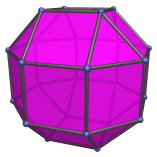There are two distinct kinds of square faces on the rhombicuboctahedron: the first kind are the axial faces which are surrounded by 4 other square faces. There are six of these faces, and they correspond with the faces of a cube: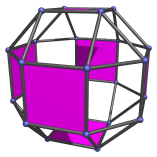The second kind of square face are the non-axial faces, which are surrounded by 2 squares and 2 triangles. There are 12 of them, and they correspond with the edges of a cube: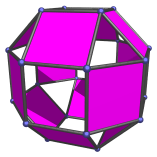It is important to distinguish between these two kinds of square faces, because their relative position to the triangular faces gives them different functions when the rhombicuboctahedron is fitted together with other polyhedra into 4D polytopes.

The dual of the rhombicuboctahedron is the deltoidal icositetrahedron, a Catalan solid.

## Projections

In order to be able to identify the rhombicuboctahedron in various projections of 4D objects, it is useful to know how it appears from various viewpoints. The following are some of the commonly-encountered views:

Projection Envelope Description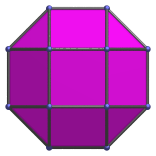Regular octagon

Parallel projection centered on an axial square face.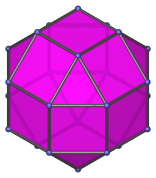Regular hexagon

Triangle-centered parallel projection.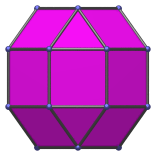Octagon

Parallel projection centered on non-axial square face.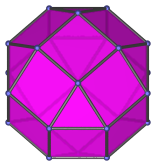Octagon

Vertex-centered parallel projection.

## Geometric Relations

The rhombicuboctahedron can be cut into two square cupolae (J4) and an octagonal prism, or into a square cupola and an elongated square cupola (J19). It can also be gyrated to form the gyroelongated square bicupola (J37). The latter has the same configuration of faces around every vertex (3 squares and a triangle), but is not vertex-transitive, hence it is not uniform, but is a Johnson solid.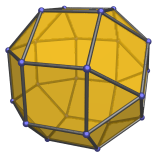## Coordinates

The Cartesian coordinates of the rhombicuboctahedron, centered on the origin and having edge length 2, are all permutations of coordinates and changes of sign of:

• (1, 1, (1+√2))

## Occurrences

The rhombicuboctahedron appears as cells in the following 4D uniform polytopes:

The rhombicuboctahedron also appears as cells in a number of CRF polytopes, including (but not limited to):

Last updated 06 Jan 2023.